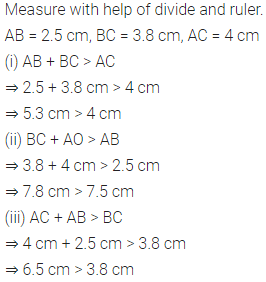ML Aggarwal Class 6 Solutions Chapter 11 Understanding Symmetrical Shapes Ex 11.1 for ICSE Understanding Mathematics acts as the best resource during your learning and helps you score well in your exams.

## ML Aggarwal Class 6 Solutions for ICSE Maths Chapter 11 Understanding Symmetrical Shapes Ex 11.1

Question 1.
Why is it better to use a divider and a ruler than a ruler only, while measuring the length of a line segment?
Solution: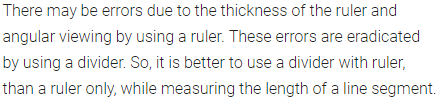Question 2.
In the given figure, compare the line segments with the help of a divider and fill in the blanks by using the symbol >, = or <:
(i) AB ………. CD
(ii) BC ………. AB
(iii) AC ………. BD
(iv) CD ………. BD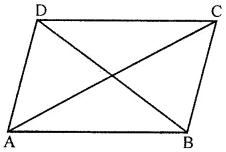Solution:Question 3.
If A, B and C are collinear points such that AB = 6 cm, BC = 4 cm and AC = 10 cm, which one of them lies between the other two?
Solution:Question 4.
In the given figure, verify the following by measurement:
(i) AB + BC = AC
(ii) AC – BC = AB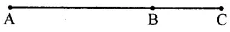Solution:Question 5.
In the given figure, verify by measurement that:
(i) AC + BD = AD + BC
(ii) AB + CD = AD – BC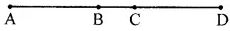Solution: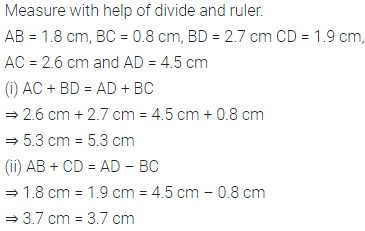Question 6.
In the given figure, measure the lengths of the sides of the triangle ABC and verify:
(i) AB + BC > AC
(ii) BC + AC > AB
(iii) AC + AB > BCSolution: